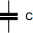# Capacitor energy charge calculator

## Calculates the amount of energy in a capacitor

 Example 1: Must calculate the energy of a 2200uF capacitor charged to 60 volts View example